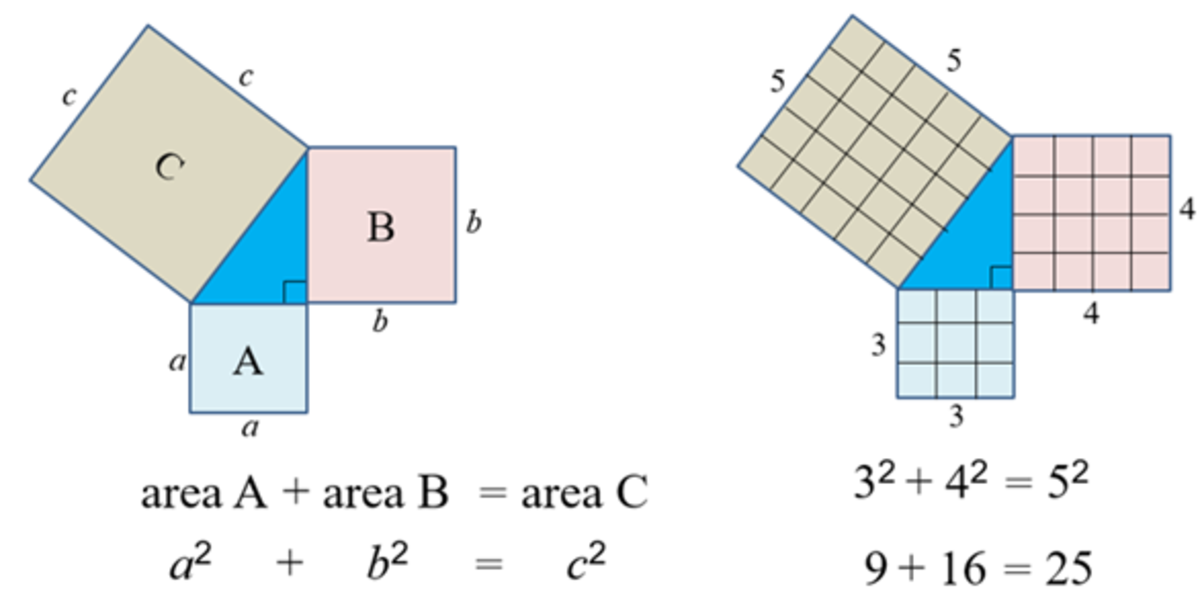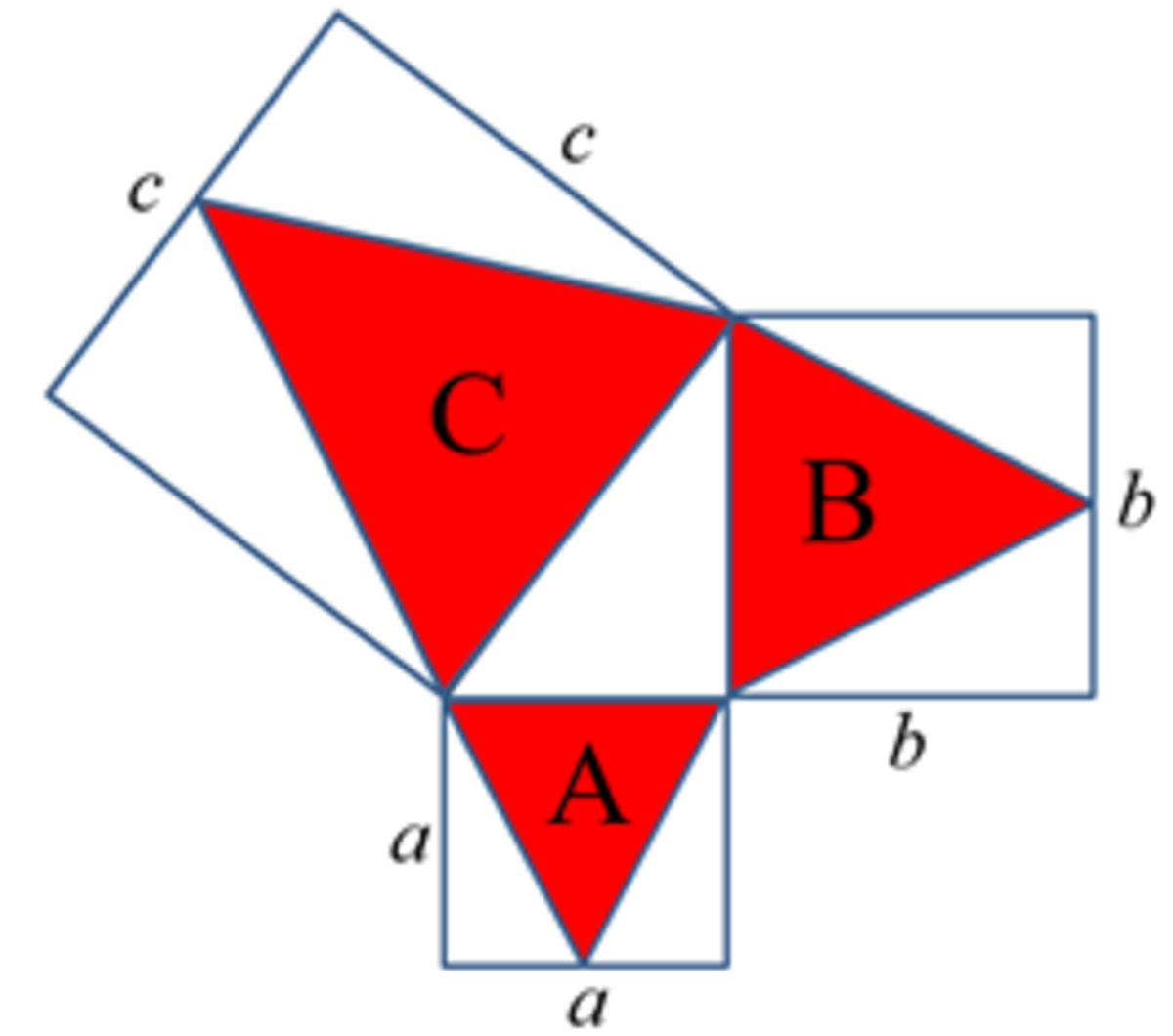# Pythagoras’ Theorem Using Polygons, Circles and Solids

I am a school teacher with a love for writing short stories, usually with a humorous twist.

## Introduction

The theorem of Pythagoras states that for a right-angled triangle with squares constructed on each of its sides, the sum of the areas of the two smaller squares is equal to the area of the largest square.The Pythagoras' Theorum was discovered more than 2000 years ago.

In the diagram, a, b and c are the side lengths of square A, B and C, respectively. Pythagoras’ theorem states that area A + area B = area C, or a2 + b2 = c2.

There are many proofs of the theorem which you might wish to investigate. Our focus will be to see how Pythagoras’ theorem can be applied to shapes other than squares, including three-dimensional solids.

## Pythagoras’ Theorem and Regular Polygons

Pythagoras’ theorem involves areas of squares, which are regular polygons.

A regular polygon is a two-dimensional (flat) shape where each side has the same length.

Here are the first eight regular polygons.

We can show that Pythagoras’ theorem applies to all regular polygons.

As an example, let’s prove that the theorem is true for regular triangles.

First, construct regular triangles, as shown below.Showing how Pythagoras’ theorem applies to regular triangles

The area of a triangle with base B and perpendicular height H is (B x H)/2.

To determine the height of each triangle, divide the equilateral triangle into two right-angled triangles and apply Pythagoras’ theorem to one of the triangles.

Scroll to Continue

## Read More From Owlcation

For triangle A in the diagram, proceed as follows.

We use the same method to find the height of the remaining two triangles.

Hence, the height of triangles A, B and C are, respectively:

The areas of the triangles are:

We know from Pythagoras’ theorem that a2 + b2 = c2.

Hence, by substitution we have the following:

Or, expanding the brackets on the left side leads us to this:

Therefore, area A + area B = area C

## Pythagoras’ Theorem With Regular Polygons

To prove the general case that Pythagoras’ theorem is true for all regular polygons, knowledge of the area of a regular polygon is required.

The area of an N-sided regular polygon of side length s is given by

As an example, let’s calculate the area of a regular hexagon.

Using N = 6 and s = 2, we have

Now, to prove that the theorem applies to all regular polygons, align the side of the three polygons with a side of the triangle, such as for the hexagon shown below.

Then we have

Therefore

But again from Pythagoras’ theorem, a2 + b2 = c2.

Hence, by substitution we have

Therefore, area A + area B = area C for all regular polygons.

## Pythagoras’ Theorem and Circles

In a similar way, we show that Pythagoras’ theorem applies to circles.

The area of a circle of radius r is πr2, where π is the constant approximately equal to 3.14.

Hence:

But once again, Pythagoras’ theorem states that a2 + b2 = c2.

Hence, by substitution we have

## The Three-Dimensional Case

By constructing rectangular prisms (box shapes) using each side of the right-angled triangle, we will show that there is a relationship between the volumes of the three cubes.

In the diagram, k is an arbitrary positive length.

Hence

volume A is a x a x k or a2k

volume B is b x b x k or b2k

volume C is c x c x k or c2k

So volume A + volume B = a2k + b2k = (a2 + b2)k

But from Pythagoras’ theorem, a2 + b2 = c2.

So volume A + volume B = c2k = volume C.

## Summary

• By constructing regular polygons on the sides of a right-angle triangle, Pythagoras’ theorem was used to show that the sum of the areas of the two smaller regular polygons is equal to the area of the largest regular polygon.
• By constructing circles on the sides of a right-angle triangle, Pythagoras’ theorem was used to show that the sum of the areas of the two smaller circles is equal to the area of the largest circle.
• By constructing rectangular prisms on the sides of a right-angle triangle, Pythagoras’ theorem was used to show that the sum of the volumes of the two smaller rectangular prisms is equal to the volume of the largest rectangular prism.

## A Challenge for You

Prove that when spheres are used, volume A + volume B = volume C.

Hint: The volume of a sphere of radius r is 4πr3/3.

This content is accurate and true to the best of the author’s knowledge and is not meant to substitute for formal and individualized advice from a qualified professional.